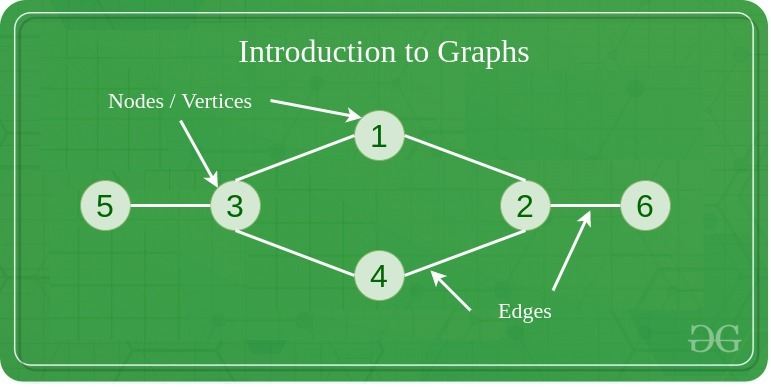# Graph Data Structure And Algorithms

• Last Updated : 08 May, 2023

## What is Graph Data Structure?

A Graph is a non-linear data structure consisting of vertices and edges. The vertices are sometimes also referred to as nodes and the edges are lines or arcs that connect any two nodes in the graph. More formally a Graph is composed of a set of vertices( V ) and a set of edges( E ). The graph is denoted by G(E, V).## Components of a Graph

• Vertices: Vertices are the fundamental units of the graph. Sometimes, vertices are also known as vertex or nodes. Every node/vertex can be labeled or unlabelled.
• Edges: Edges are drawn or used to connect two nodes of the graph. It can be ordered pair of nodes in a directed graph. Edges can connect any two nodes in any possible way. There are no rules. Sometimes, edges are also known as arcs. Every edge can be labeled/unlabelled.

Graphs are used to solve many real-life problems. Graphs are used to represent networks. The networks may include paths in a city or telephone network or circuit network. Graphs are also used in social networks like linkedIn, Facebook. For example, in Facebook, each person is represented with a vertex(or node). Each node is a structure and contains information like person id, name, gender, locale etc.

Topics:

Introduction:

BFS and DFS in Graph:

Cycles in Graph:

Shortest Path in Graph:

Minimum Spanning Tree:

Topological Sorting:

Connectivity:

Maximum Flow

Some must do Problems on Graph:

Some Quizzes: